Next: Dyadic Rational Streams Up: Representing Reals as Streams Previous: Representing Reals as Streams

## Signed Binary Digit Streams

The first representation makes use of a stream of signed binary digits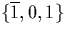. This representation is also known as the redundant binary representation on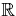. The real number represented by the stream x = (d1::d2::d3::...) is defined as: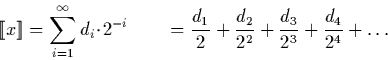Hence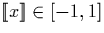. There is a unique representation for the numbers minus one (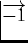) and one (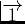), and infinitely many representations for all other numbers in this range.

We also define the range (see section 3.1) of a finite portion of the stream as follows: Let x' be a list containing the first m digits of x,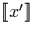is the range of x':

Figure 3.1 shows the range of values represented by the signed binary streams starting with each of the three possible digits.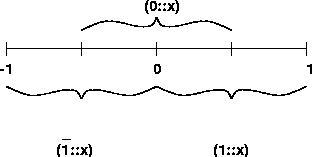There are two further interesting observations to make. The first is that the following identities exist. We use them frequently when developing algorithms using signed binary streams:

The second observation is that the signed binary representation has a fixed rate of convergence. In other words, every new digit in the stream refines the range of possible values taken by the stream at a fixed rate.Next: Dyadic Rational Streams Up: Representing Reals as Streams Previous: Representing Reals as Streams
Martin Escardo
5/11/2000